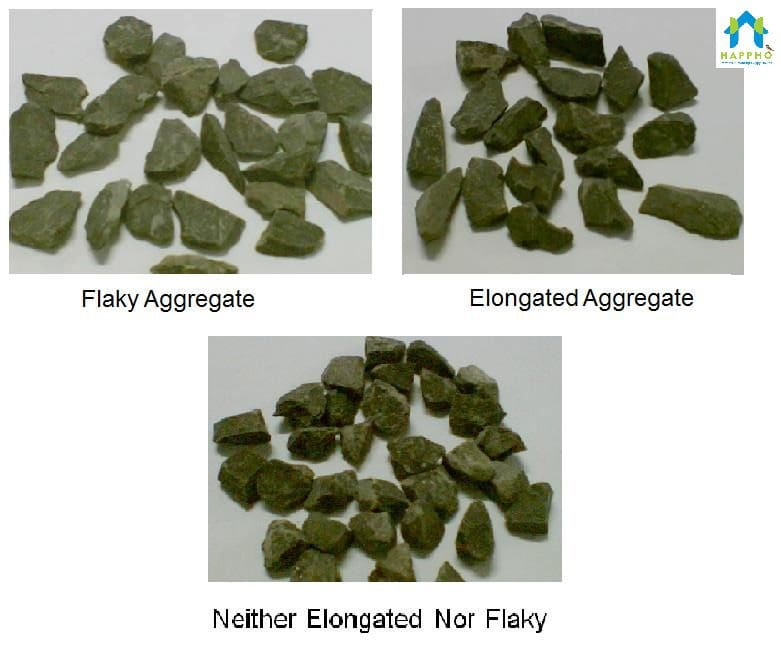# How Cement, Sand and Aggregate ratios influence Concrete Properties?

Aggregate to Cement ratio is important as they are used to arrive at optimized, workable and cohesive concrete for a set of locally available concrete ingredients.

In this context, the total Aggregate mean the sum of weights of Sand (Fine Aggregate) and Aggregate (usually called Coarse Aggregate)

While analyzing/designing a concrete mix, Aggregate cement ratio plays following important roles.

1. Total Aggregate by cement ratio (A/C) decreases with increase in grade of concrete. For example, M20 grade of aggregate will have a higher A/C ratio than of M30

Higher the Aggregate/Cement ratio leaner the concrete mix, as cement content is lower.

1. Concrete mix can be made more workable by fine tuning Aggregate/Cement ratio. The same is governed by particle size and shape of aggregate used. A flaky and elongated aggregate will demand higher cement content (as they have higher surface area for the same volume occupied, requiring higher cement paste). On the other hand, an angular aggregate will require lower cement to achieve at par work abilityAlso, while analyzing/designing a concrete mix, Sand to total Aggregate ratio plays following important roles

1. In total aggregate, the proportion of sand to coarse aggregate (C.A) is altered depending on fineness of sand
2. A fine sand reduces the sand requirement % in the total aggregate proportion. On the other hand, a coarse sand will require higher sand % in the total aggregate proportion to give a cohesive concrete mix